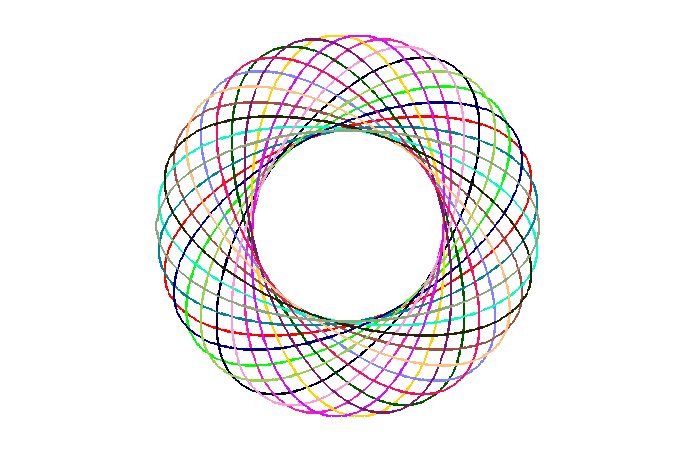Drawing Ellipses4

Posted by Brett Shoelson,

Brett's Pick this week is ellipse, by David Long.

I've blogged before about fitting circles, and about simply drawing circles. And I've blogged about fitting ellipses. I like the general utility of functionality that facilitates those common tasks. Today, I complete the suite with a Pick that trivializes the drawing of an ellipse.

David's syntax is quite nice:

h=ellipse(ra,rb,ang,x0,y0,C,Nb);

There are defaults for all of the inputs (you'd get a circle of radius 1, plotted at [0,0]), but most usefully, you would specify, at a minimum, the semi-major (ra) and semi-minor (rb) axes lengths, the desired angle, and the coordinates of the center (plus, optionally, the color and the number of points used in the construction).

Remember Spirograph? Here's a fun little graphic I created using David's ellipse function, and another previous pick, distinguishable_colors:

colors = distinguishable_colors(20);
n = 20;
angles = linspace(0,2*pi,n);
h = zeros(n,1);
for ii = 1:n
h(ii) = ellipse(2,1,angles(ii),0,0,colors(ii,:));
end
axis equal
axis off
set(h,'linewidth',2)
set(gcf,'color','w')As always, comments to this blog post are welcome. Or leave a comment for David here.

Get the MATLAB code

Published with MATLAB® 7.13

18 views (last 30 days)  | |